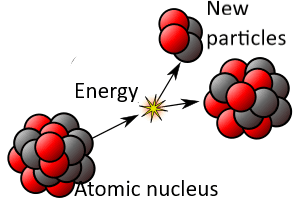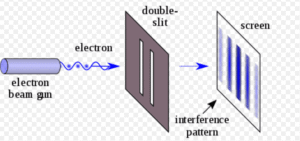Home » quantum randomness

# quantum randomness

« Back to Glossary Index

Many quantum physicists view quantum mechanics as differing from classical physics in a fundamental way—quantum mechanics is not deterministic. It is beset by “true randomness.” At the same time other physicists view quantum mechanics as deterministic. While there is considerable agreement among physicists on the mathematics and experimental results of quantum mechanics, there’s less agreement on how to interpret these, including in regard to determinism.Schematic of radioactive decay of a generic atomic nucleus[Revision of the original image by Kjerish – Own work, CC BY-SA 4.0, https://en.wikipedia.org/wiki/Radioactive_decay]Most of the early interpretations of quantum mechanics include the principle of quantum randomness, a principle that violates determinism. Consider the example of the moment when a radioactive atom of Uranium 235 decays. To say that the atom decays is to say that its nucleus abruptly loses energy and transforms into other particles. This is the Weak Force at work. Radioactive decay becomes more likely as time passes.*

Even though each atom is identical, the time required for decay varies among atoms, apparently randomly. What triggers the Weak Force to become active and the nucleus to emit energy and transform into other particles? Physicists cannot assign a cause to that. It is as though each atom chooses its own moment. (For more, see the entry Free Will Theorem.)

In contrast, in classical physics, nothing happens randomly. If a billiard ball rolls to the right, it’s because some force is pushing it in that direction. If we don’t know the nature of the force, it might seem like it’s random motion. But physicists tell us, rest assured, there is a prior causal force. The appearance of randomness is only a comment on our ignorance. This is to say that the mathematics of classical physics is deterministic—every physical action is determined by prior actions.

In the “true randomness” of quantum mechanics, we don’t know the cause and, also, many physicists say that there is none.

## Quantum Randomness in Experimental ResultsDouble slit experiment with electrons [Image source: https://en.wikipedia.org/wiki/Double-slit_experiment]When doing the Double Slit Experiment, physicists shoot a particle, let’s say an electron, through slits in a barrier towards a detection screen. Physicists use the Schrodinger Equation to predict where the electron will land on the detection screen. But the equation predicts only probabilities, not the position in which any particular electron will land. This is similar to being able to predict the half-life of a group of uranium atoms but not the moment at which any particular uranium atom will decay.

If the Double Slit Experiment is repeated numerous times, perhaps thousands of times, the results will reflect the probabilities of the Schrodinger Equation. For example, if the equation calculates a 50% probability for any particular position, 50% of electrons will be found to land in that position. However, physicists are unable to predict the position of any particular electron; the equation is silent on that question.

## Quantum Randomness in Theory

Schrodinger’s Equation is a key equation of quantum mechanics. It, and the equations which were built upon it, have been extremely successful at predicting the behavior of quantum particles. Every day, physicists and engineers work with electronics on the assumption that quantum particles act, in certain ways, randomly but within the constraints of probability. Using the probabilistic equations of quantum mechanics, physicists have developed the modern electronics which have revolutionized our world: computers, cell phones, etc. So, physicists are confident of these equations. Yet, in many interpretations, the equations are not deterministic of individual particle behavior.

## Could quantum particles be influenced by hidden variables?

The original interpretation of quantum mechanics, the Copenhagen Interpretation, assumes true randomness. While most later interpretations do as well, the Bohmian interpretation does not. David Bohm developed Bohmian Mechanics in the 1950’s. The equations of Bohmian Mechanics are the same as those of other forms of quantum mechanics except that Bohm added an additional equation.